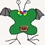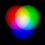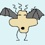type="text/css" />

### New Game: Multiplier General forum

9 replies. Last post: 2021-10-09

New Game: Multiplier
•simpledeep at 2021-10-08

I tried to find a mathematical combinatorial game, and I wanted it to be a Pen-&-Paper game too. This is what I came up with:

Here is my initial analysis of the game:

If you scroll down in that thread you will see that someone has already brute-forced 3x3 for us, and it looks like pie should work nicely. I'm still not sure which player may or may not have an advantage on 4x4, and I'm not sure what the best way to implement pie for a game with second player advantage would be.

Draws should be pretty rare, but they are out there! If my feelings about advantage turn out to be correct, then I suppose it makes sense to award draws to the player who's most likely to be at a disadvantage right?

You can easily test the game for yourselves in “google sheets”:

•Enforce Aspiration of Vaccine at 2021-10-08

It all adds up to a draw sometimes?, any examples

•Enforce Aspiration of Vaccine at 2021-10-08

7 2 9 126
4 5 8 160
1 6 3 18 304
28 60 216

``````    304     got one
``````
•Enforce Aspiration of Vaccine at 2021-10-09

Instead of a series of whole number

Can a series of prime numbers produce a draw?

•simpledeep at 2021-10-09

I wondered this myself. So I tried the pattern I found that provided a draw on every regular sequence AND the only-odds sequence I tried. I used the equivalent pattern but with prime numbers 2-23 and it was NOT a draw. Of course that in no way proves that draws are impossible with only-primes like that.

I still haven't even been able to find a single draw 4x4 yet…

•gamesorry at 2021-10-09

4x4 draws seem to be quite possible:

[[x, 2, 3, 4],
[8, 7, 6, 5],
[9, 10, 11, 12],
[1, 15, 14, y]]

x = 16, y = 264, sum_row = 69384, sum_col = 69384
x = 21, y = 256, sum_row = 67824, sum_col = 67824
x = 26, y = 248, sum_row = 66264, sum_col = 66264
x = 31, y = 240, sum_row = 64704, sum_col = 64704
x = 36, y = 232, sum_row = 63144, sum_col = 63144
x = 41, y = 224, sum_row = 61584, sum_col = 61584
x = 46, y = 216, sum_row = 60024, sum_col = 60024
x = 51, y = 208, sum_row = 58464, sum_col = 58464
x = 56, y = 200, sum_row = 56904, sum_col = 56904
x = 61, y = 192, sum_row = 55344, sum_col = 55344
x = 66, y = 184, sum_row = 53784, sum_col = 53784
x = 71, y = 176, sum_row = 52224, sum_col = 52224
x = 76, y = 168, sum_row = 50664, sum_col = 50664
x = 81, y = 160, sum_row = 49104, sum_col = 49104
x = 86, y = 152, sum_row = 47544, sum_col = 47544
x = 91, y = 144, sum_row = 45984, sum_col = 45984
x = 96, y = 136, sum_row = 44424, sum_col = 44424
x = 101, y = 128, sum_row = 42864, sum_col = 42864
x = 106, y = 120, sum_row = 41304, sum_col = 41304
x = 111, y = 112, sum_row = 39744, sum_col = 39744
x = 116, y = 104, sum_row = 38184, sum_col = 38184
x = 121, y = 96, sum_row = 36624, sum_col = 36624
x = 126, y = 88, sum_row = 35064, sum_col = 35064
x = 131, y = 80, sum_row = 33504, sum_col = 33504
x = 136, y = 72, sum_row = 31944, sum_col = 31944
x = 141, y = 64, sum_row = 30384, sum_col = 30384
x = 146, y = 56, sum_row = 28824, sum_col = 28824
x = 151, y = 48, sum_row = 27264, sum_col = 27264
x = 156, y = 40, sum_row = 25704, sum_col = 25704
x = 161, y = 32, sum_row = 24144, sum_col = 24144
x = 166, y = 24, sum_row = 22584, sum_col = 22584
x = 171, y = 16, sum_row = 21024, sum_col = 21024

•gamesorry at 2021-10-09

Found an all-prime solution for 4x4 draw:

[[359, 5, 19, 2],
[23, 37, 43, 17],
[29, 47, 31, 7],
[3, 11, 13, 823]]

sum_row = 1339129, sum_col = 1339129

•simpledeep at 2021-10-09

That's cool, but it appears that each of your examples involves one or two numbers from the set being wildly different from the rest. I think it might ruin the gameplay if you ever tried actually playing a match with any of the sets used in these examples.

Playing with just primes might be ok if its something like 2-53 or 29-97 for example. That way you wouldn't have any one or two numbers that stand out as completely overpowered compared to the rest.

Then again, it's such a simple game anyways, it may be just as fun to use rng for all I know! :P

Did you use a computer to search for these? If so did you try to find draws for more “normal” sets like 1-16 or 3-18? Just what kind of computational resources would it take to search for every possible score of a given number-sequence on 4x4?

•simpledeep at 2021-10-09

Is it even possible to make an educated guess on whether draws are more or less likely on 4x4 compared to 3x3?